Homework Help Question & Answers

# 13. Write the equilibrium constant equation for the following reversible reactions.(3 points for the first two...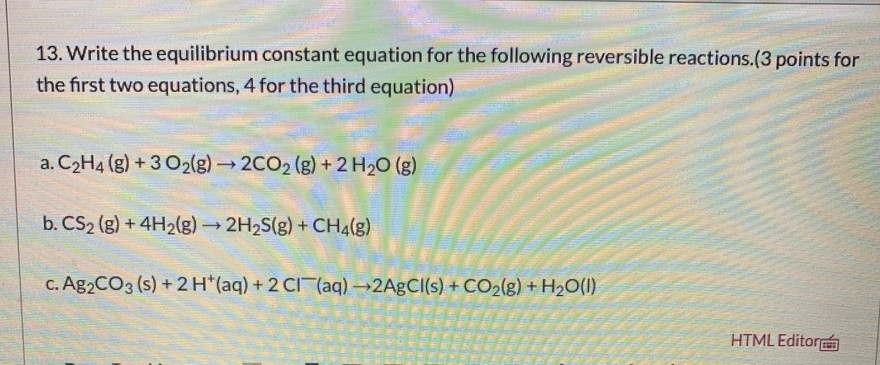13. Write the equilibrium constant equation for the following reversible reactions.(3 points for the first two equations, 4 for the third equation) a. C2H4 (g) + 3 O2(g) → 2CO2 (g) + 2 H20 (g) b. CS2 (g) + 4H2(g) + 2H2S(g) + CH4(E) c. Ag2CO3 (5) + 2H*(aq) + 2 C1(aq) —2AgCl(s) + CO2(g) + H20(1) HTML Editora

#### Homework Answers

Answer #1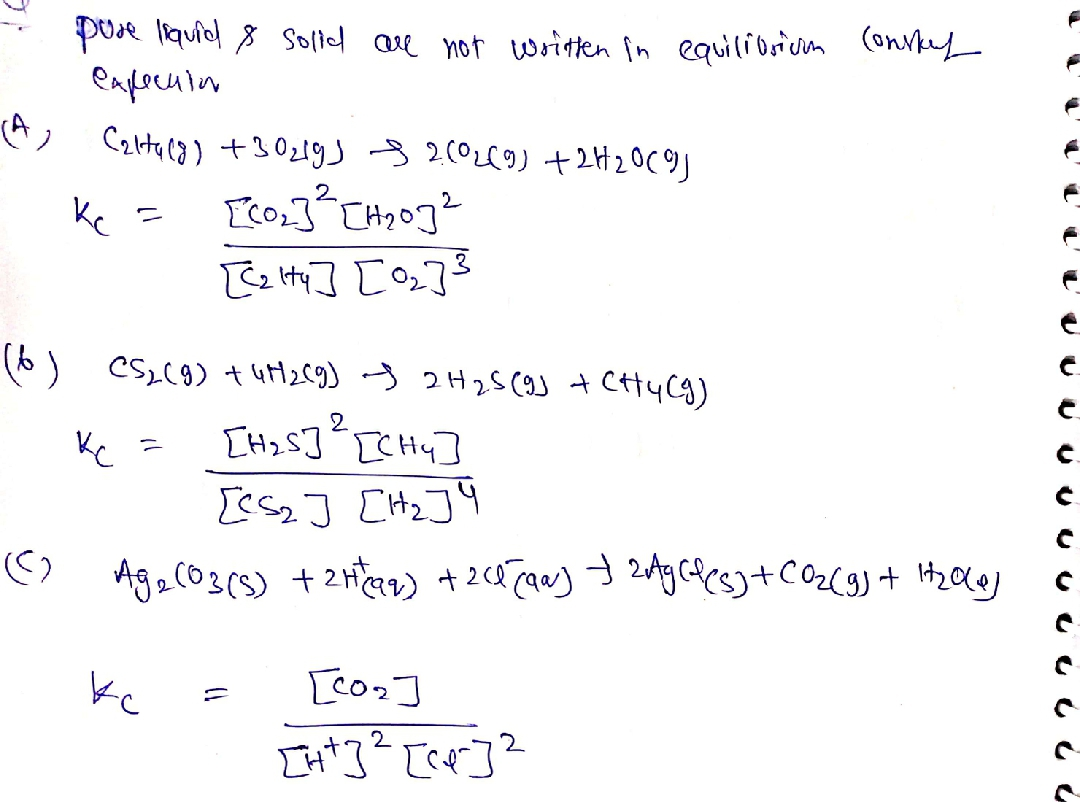Know the answer?
Your Answer:

#### Post as a guest

Your Name:

What's your source?

#### Earn Coin

Coins can be redeemed for fabulous gifts.

Not the answer you're looking for? Ask your own homework help question. Our experts will answer your question WITHIN MINUTES for Free.
Similar Homework Help Questions
• ### What are the equilibrium constant expressions (Kc) for the following reactions

1. Write the equilibrium constant expressions (Kc) for the following reactions: (a)  CO (g)  +  H2O (g)   ⮂   CO2 (g)  +  H2 (g) (b)  CH4 (g)  +  2H2S (g)   ⭢   CS2 (g)  +  4H2 (g) (c)  COCl2 (g)   ⮂   Cl2 (g)  +  CO (g) (d)  2HI (g)   ⮂   H2 (g)  +  I2 (g) (e)  PCl3  +  Cl2 (g)   ⮂   PCl5 (g) (f)  2H2 (g)  +  O2 (g)   ⮂  ...

• ### Estimate the value of the equilibrium constant at 510 K for each of the following reactions....

Estimate the value of the equilibrium constant at 510 K for each of the following reactions. a) 2CO(g)+O2(g)⇌2CO2(g) b) 2H2S(g)⇌2H2(g)+S2(g) There was no additional information given.

• ### 3. You are given the following data for the following reversible reaction that is considered elementary....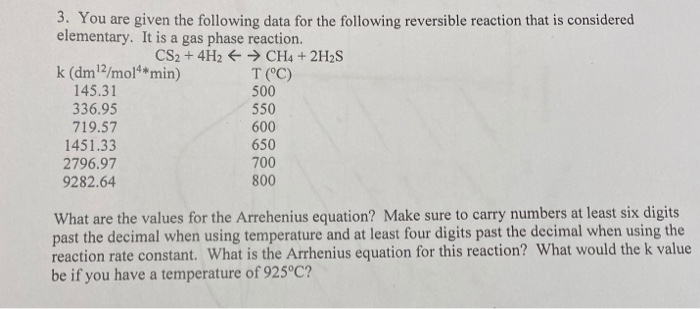3. You are given the following data for the following reversible reaction that is considered elementary. It is a gas phase reaction. CS2 + 4H2 → CH4 + 2H2S k (dm2/mol** min) T(°C) 145.31 500 336.95 719.57 600 1451.33 650 2796.97 700 9282.64 800 550 What are the values for the Arrehenius equation? Make sure to carry numbers at least six digits past the decimal when using temperature and at least four digits past the decimal when using the reaction...

• ### 2) Write the equilibrium constant expressions for each of the following reactions. (For gas-phase reactions, write...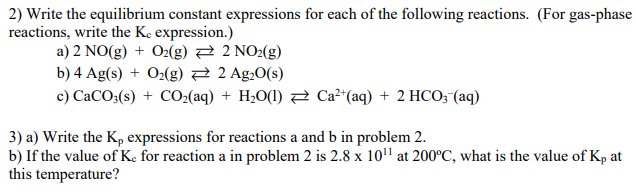2) Write the equilibrium constant expressions for each of the following reactions. (For gas-phase reactions, write the Ke expression.) a) 2 NO(g) + O2(g) = 2 NO2(g) b) 4 Ag(s) + O2(g) + 2 Ag2O(S) c) CaCO3(s) + CO2(aq) + H2O(l) = Ca2+(aq) + 2 HCO3(aq) 3) a) Write the K, expressions for reactions a and b in problem 2. b) If the value of K for reaction a in problem 2 is 2.8 x 1011 at 200°C, what is...

• ### a) Balanced the following molecular equations for these reactions. b) Write the ionic equation for the...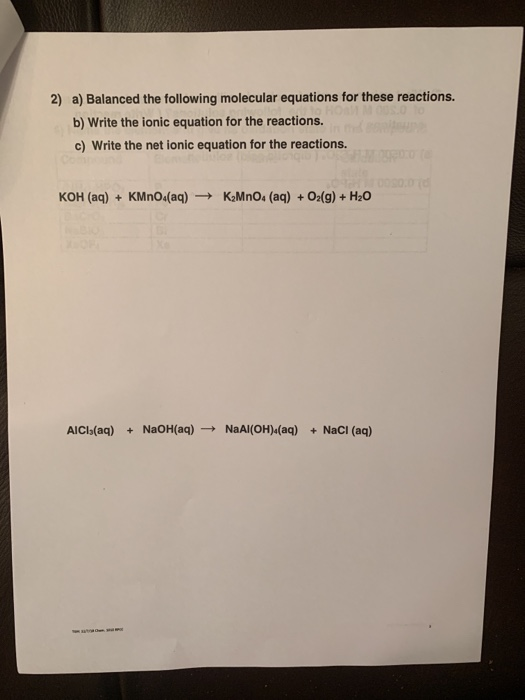a) Balanced the following molecular equations for these reactions. b) Write the ionic equation for the reactions. c) Write the net ionic equation for the reactions. 2) KOH (aq) KMno4(aq)K2MnOs (aq)+O2(g) + H20 AICI (aq) + NaOH(aq) NaAI(OH)4(aq) + NaCI (aq)

• ### Review 1: Equilibrium 1. Write equilibrium expressions for the following reversible reactions: a. 2 NO: (g=N.O.(...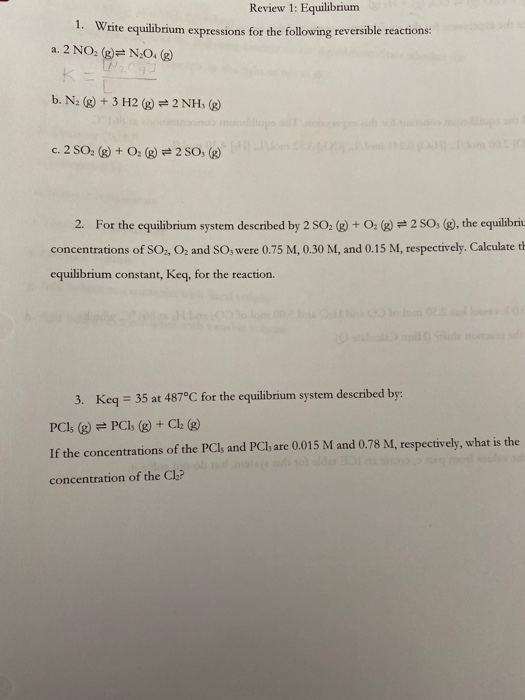Review 1: Equilibrium 1. Write equilibrium expressions for the following reversible reactions: a. 2 NO: (g=N.O.( b. N. (g) + 3 H2(g) = 2 NH, (g) c. 2 SO, (g) + O2(g) = 2 SO, (g) 2. For the equilibrium system described by 2 SO2(g) + O(g) = 2 SO, (g), the equilibrio concentrations of SO, O, and SO, were 0.75 M, 0.30 M, and 0.15 M, respectively. Calculate th equilibrium constant, Keq, for the reaction. 3. Keq = 35...

• ### Write the equilibrium constant expressions for the follow- ing reactions: (a) 2 CO(g) + O2(g) 2...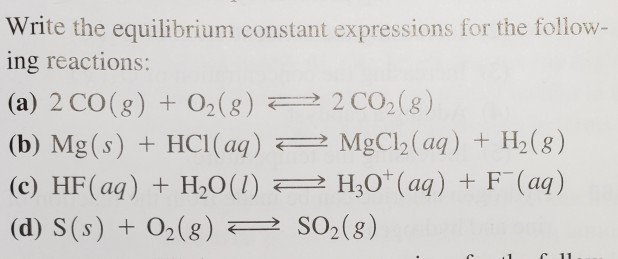Write the equilibrium constant expressions for the follow- ing reactions: (a) 2 CO(g) + O2(g) 2 2 CO2(8) (b) Mg(s) + HCl(aq) 2 MgCl2(aq) + H2(8) (c) HF(aq) + H2O(l) 2 H30+(aq) + F(aq) (d) S(s) + O2(8) 2 SO2(8) e 1 11

• ### 11. It is possible to measure the enthalpy change for the two reactions below. Reaction equation...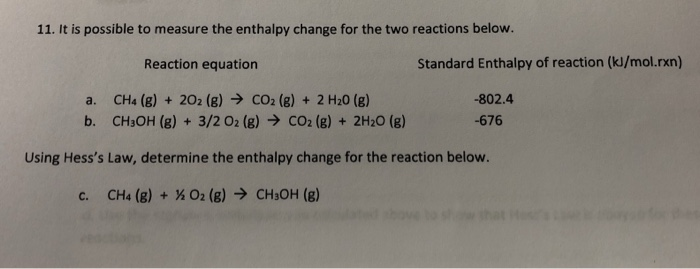11. It is possible to measure the enthalpy change for the two reactions below. Reaction equation Standard Enthalpy of reaction (kJ/mol.rxn) a. CH4 (g) + 2O2 (g) → CO2(g) + 2 H20 (g) b. CH3OH (g) + 3/2 02(g) → CO2(g) + 2H20 (8) -802.4 -676 Using Hess's Law, determine the enthalpy change for the reaction below. C. CH4 (8) + % O2 (8) > CH3OH (g)

• ### Write equilibrium constant expressions for the following reactions in terms of concentration: (a) 2 CH C12(E)=...Write equilibrium constant expressions for the following reactions in terms of concentration: (a) 2 CH C12(E)= CH (8) + CC14(E) (6) Ti(s) + 2 H2O(g)— 1102() + 2 H2(g) (°) HC H20c(aq) + H20(1)=H20*(aq) + CH 06 (aq) Check & Submit Answer Show Approach

• ### Solve these two questions plz ASAP 6. Consider the chemical equation and equilibrium constant for the...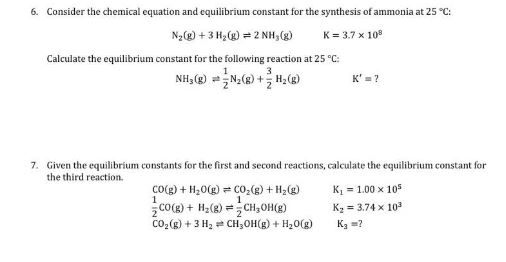Solve these two questions plz ASAP 6. Consider the chemical equation and equilibrium constant for the synthesis of ammonia at 25 °C: Ng(g) + 3 H2(g-2NH3(g) K=3.7 x 108 Calculate the equilibrium constant for the following reaction at 25 C: 7. Given the equilibrium constants for the first and second reactions, calculate the equilibrium constant for CO2 (g) + H2(g) CH3OH(g) the third reaction. K, = 1.00× 105 co(g) + H2O(g) 2co(g) + H2(g) K2 3.74x 103